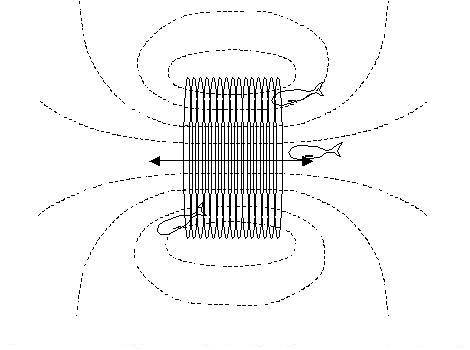# Mutual inductance question

McLaren Rulez

## Homework Statement

One solenoid is centered inside another, The outer one has a length of 50cm and has 6750 coils, while the coaxial inner solenoid is 3cm long and 0.12cm in diameter and contains 15 coils. What is the mutual inductance of these solenoids?

## Homework Equations

M=N1$$\phi$$1/I2

M=N2$$\phi$$2/I1

Note: 1 refers to the outer loop and 2 the inner loop.

## The Attempt at a Solution

I used both the above equations. One way is to find the B1 due to the outer solenoid, multiply that B1 by the area of the inner solenoid to get the flux through coil of the inner solenoid, $$\phi$$2. Then use the second equation. But that answer which is (2.8*10^-7)H is incorrect.

Then I tried multiplying B2 with the area of the inner solenoid again to get $$\phi$$1. Then I used the first equation to get the answer which is (4.8*10^-6)H and this is correct.

Why is the first method wrong? This seems to be giving me two different values for the mutual inductance between two coils. Thank you for your help! If it helps, this is taken from the textbook University Physics by Young and Freedman. It is problem 30.43 in edition 12.

Last edited:

McLaren Rulez
Bump! Can anyone shed some light please?

Antiphon
Yes. You are using formulas that don't apply to your problem.

One solenoid is very long while the other is very short. When you use the formula for the short solenoid, all of it's turns link with flux from the long solenoid. But the other formula has a huge number of turns. The flux from the distant turns of the long solenoid don't link the turns of the smaller solenoid.

McLaren Rulez

Could you elaborate on what you mean by linking flux? I didn't quite get what you said.

Let me tell you what I am thinking. I don't understand why the flux from the distant turns matter here. Shouldn't it be that we simply look at the the number of turns per unit length of the outer solenoid and thus find the magnitude of the B field that it produces, then take the area of the inner solenoid to get the flux?

And on the same note, the method which yielded the correct answer seems rather wrong to me. The inner solenoid's flux looks like the image here, yes? (Please ignore the stupid fish that somehow got in here :D)So if there's an outer solenoid around this guy that's stretching much further, wouldn't it be incorrect to say that the flux through the distant coils of the outer solenoid is the same as the flux through the nearby coils?

McLaren Rulez